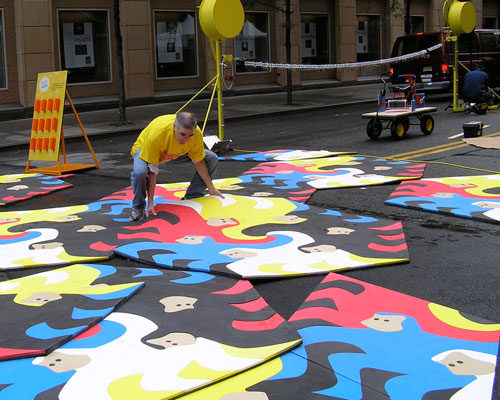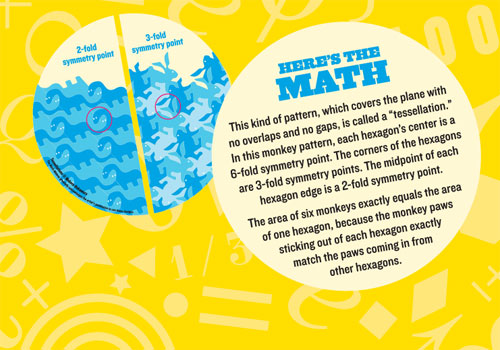Activities - Mathematical Monkey Mat

Look at the symmetry of the mat. A 3-fold symmetry point is a spot where the pattern can be rotated one third of the way around and still look the same (although the colors change). Can you find 3-fold symmetry points in the pattern? How about 180 degree 2-fold symmetry points?This kind of pattern, which covers the plane with no overlaps and no gaps, is called a "tessellation." In this monkey pattern, each hexagon's center is a 6-fold symmetry point. The corners of the hexagons are 3-fold symmetry points. The midpoint of each hexagon edge is a 2-fold symmetry point.